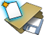# Tutorial: Simulating a Hybrid Model

Learn how to model and simulate a simple hybrid system that includes discrete signals and continuous linear systems.

## Files for This Tutorial

HybridSystem.scm

A finished version of the models you build in the tutorials along with any files required to complete the tutorials are available at this location: <installation_directory>/tutorial_models/.

## Overview

The model in this tutorial includes DiscreteDelay blocks to generate a square-wave signal that is fed into the input of a continuous-time linear system. The continuous-time linear system is represented by its transfer function via a ContTransFunc block. A Scope block plots the output of the system.

Constant-coefficient linear differential equations can be expressed in Laplace domain by applying the Laplace transform to both sides of the equation. This operation transforms the differential equation into an algebraic equation since the differentiation operation is converted to multiplication by the independent variable, s. If the differential equation represents the input-output behavior of a system in the time domain, the resulting equation in the Laplace domain can be used to obtain the system’s transfer functions. For example, the linear differential equation: yields the transfer function: The ContTransFunc block can represent a system’s single-input, single- or possible multi-output, transfer function. The block parameters contain the coefficients of the transfer function’s denominator and numerator(s). A special case of the transfer function is an integrator, such as represented by the Integral block. In discrete-time, transfer functions are obtained by applying the Z transform to difference equations. The special case of a unit delay operator is represented by the DiscreteDelay block. This block is usually activated periodically by a SampleClock block, which fixes the period and phase of the activations. Discrete-time signals are updated at activation times and remain constant from one activation to the next.

## Constructing a Discrete Signal

Construct discrete signals with the DiscreteDelay block.

1. From the ribbon, clickor, from the menu bar, click File > New.
2. Save your model as HybridSystem_practice.scm.
3. From the Palette Browser, drag and drop the following blocks into your diagram:
• From Activate > Dynamical, drag and drop two DiscreteDelay blocks into your diagram.
• From Activate > ActiveOperations, drag and drop one SampleClock block into your diagram.
4. Assemble and connect the blocks in your diagram as you see in the following figure:
5. On the SampleClock block, double-click. In the block dialog, for the Sample period, enter: 1/20000, and click OK.
6. On the block, DiscreteDelay, double-click. In the block dialog, for Initial Condition, enter: 1, and click OK.

The discrete signal is constructed as you see in the following figure. The signal generates a square wave signal toggled between 0 and 1 at the frequency of 10000Hz.

## Creating a Continuous Transfer Function

Represent continuous linear systems with the ContTransFunc block.

1. In the Palette Browser, double-click Activate > Dynamical, and drag and drop a ContTransFunc block into the diagram.
2. On the block, ContTransFunc, double-click. In the block dialog, for Coefficients of the numerator polynomial, enter: 1. For Coefficients of the denominator polynomial, enter: [0.2 1], and click OK.
The ContTransFunc block represents a first order linear system that models the behavior of a simplified motor dynamics.
3. Connect the block to your diagram as you see in the following figure:
4. In the Palette Browser, double-click Activate > SignalViewers, and drag and drop one Scope block into the diagram.
5. On the Scope block, double-click. For Number of inputs, enter: 2.
Two input ports appear on the Scope block.
6. Connect the Scope block to the diagram as you see in the following figure:

## Simulating the Hybrid Model

Set simulation parameters and run a simulation.

1. On the ribbon, select the Setup tool.
2. On the Simulation Parameters dialog, enter 2 for Final time, then click OK.
3. On the ribbon, click Run .
The simulation of the diagram begins.

## Reviewing Simulation Data

Examine the data in the plots generated from the Scope block.

1. On the Scope block, double-click.
A Scope window opens. Because the Scope block is defined with two inputs, two corresponding subplots are displayed, representing the square wave signal and system response, respectively. Because the frequency of the square wave is 10,000Hz, too many periods exist in the time span between 0 and 2 seconds, therefore zooming into a smaller range is required.
2. To reveal the square wave form more clearly, on the X-axis of the upper subplot, right-click to launch a floating panel.
3. For the upper limit value, enter 0.001.
4. On the Y-axis of the upper subplot, right-click. In the floating panel, for the lower-limit value, enter: -2; for the upper limit value, enter: 2.
5. To fit the view of the plot in the window, on the lower subplot, middle-click.
6. To zoom in on an area in the plot, press the Ctrl key + middle-click + drag to select a frame on the plot.
The area in the frame appears in the lower plot as you see in the following image:
7. To reveal specific data points on the curve, press and hold the Ctrl key while positioning your cursor on the curve.
The data points closest to the cursor are displayed.